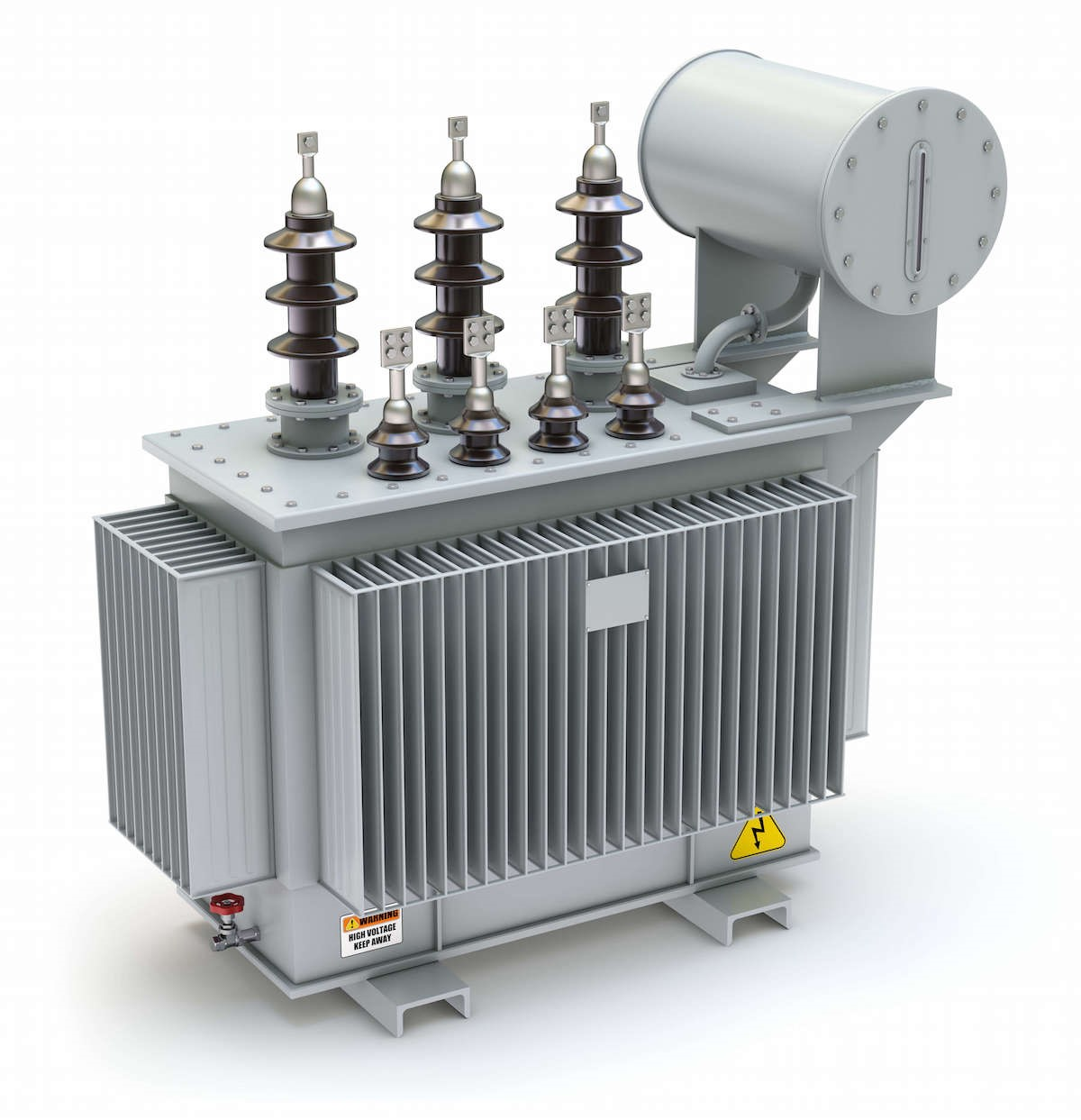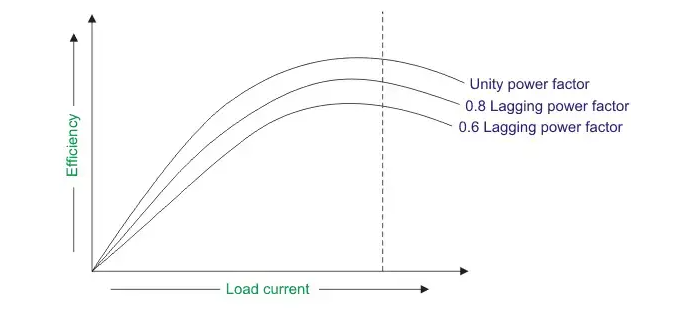Contact Number +90 536 226 00 45

## Efficiency of TransformerTransformer efficiency is achieved when the magnetic coupling between the primary and secondary windings is used in the best possible way. Efficiency indicates how much of the electrical energy supplied to the transformer is converted into output. Low efficiency leads to energy loss.Factors to consider when designing transformers are:

1. Quality of magnetic flux: Magnetic flux is the most important factor that affects transformer efficiency. The magnetic flux should be high and of high quality.
2. Quality of winding: The quality of the winding affects transformer performance. A properly and tightly wound winding increases magnetic flux and reduces losses.
3. Quality of materials: The core and windings are the main materials of the transformer. High-quality materials increase efficiency by maintaining magnetic flux and reducing losses.
4. Size: Transformer size is also a factor that affects efficiency. Larger transformers may be more efficient, but they are also more expensive and heavier.
5. Frequency: Transformer design is dependent on the frequency of use. Transformers designed for higher frequencies may be smaller and lighter, but they may also be more expensive.

Efficiency is obtained by the amount of energy output in a unit time at the nominal power of the transformer compared to the input. Ideal transformer efficiency can be 100%, but in practice, efficiency is not always at this level due to losses.

For example, assuming a 1 kVA transformer is operating at its nominal power and we measure 990 W of power at its output. The efficiency of the transformer can be calculated as follows:

Efficiency = (output power / input power) x 100%
Efficiency = (990 W / 1000 W) x 100%
Efficiency = 99%

By improving the quality of the magnetic flux, winding the windings tighter, using quality materials, optimizing the size and frequency of the transformer, and paying attention to other design factors, the transformer’s efficiency can be improved.We can see from the figure that the maximum efficiency occurs at the unity power factor. And the maximum efficiency occurs at the same loading irrespective of the power factor of the load.

In summary, transformers form the most important link between supply systems and load. Transformer’s efficiency directly affects its performance and aging. The transformer’s efficiency, in general, is in the range of 95 – 99 %. For large power transformers with very low losses, the efficiency can be as high as 99.7%. The input and output measurements of a transformer are not done under loaded conditions as the wattmeter readings inevitably suffer errors of 1 – 2%. So for the purpose of efficiency calculations, OC and SC tests are used to calculate rated core and winding losses in the transformer. Core losses depend on transformer rated voltage. Copper losses depend on the currents passing through the primary and secondary windings of the transformer. Hence transformer efficiency is of prime importance to operating it under constant voltage and frequency conditions. The rise in the temperature of the transformer due to the heat generated affects the life of transformer oil properties and decides the type of cooling method adopted. The temperature rise limits the rating of the equipment.